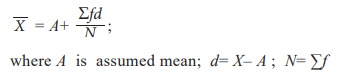# Average

Arithmetic mean of a set of observations is their sum divided by the number of observations.

Average

- Recall

There are several measures of central tendency for the data. They are

┬Ę        Arithmetic Mean

┬Ę        Median

┬Ę        Mode

┬Ę        Geometric Mean

┬Ę         Harmonic Mean

## Arithmetic Mean (discrete case)

Arithmetic mean of a set of observations is their sum divided by the number of observations. The observation are classified into a) Ungrouped data and b) Grouped data.

### (i)      Direct Method:whereis Arithmetic Mean, ŌłæX is sum of all the values of the variable X and n is number of observations.

### (ii)  Short-cut method

The arithmetic mean can be calculated by using any arbitrary value A as origin and write d as the deviation of the variable X then,### Direct method

The formula for computing the mean iswhere f is frequency; X is the variable; N = Ōłæ f i.e. total frequency.

### (ii)  Short-cut method

The arithmetic mean is computed by applying the following formula:## Arithmetic mean for Continuous case

The arithmetic mean may be computed by applying any of the following methods:

(i) Direct method

(ii) Short-cut method

(iii) Step deviation method

### (i) Direct method

When direct method is used arithmetic mean is defined asWhere m = midpoint of each of the class interval,

f = the frequency of each class interval

N = Ōłæf = total frequency

### (iii) Short-cut method

The arithmetic mean is computed by applying the following formulawhere A is assumed mean or arbitrary value, d=mŌĆōA is deviations of mid-point from assumed mean and N=Ōłæf

### (iii) Step Deviation Method

In case of grouped or continuous frequency distribution, the arithmetic meanis any arbitrary value or assumed mean and c is the magnitude of class interval.

### Mode:

Mode is the value which repeats maximum number of times among the given observations.

### Median:

Median is exactly a middle value and it exceeds and exceeded by the same number of observations. Median is one of the positional measure. Some other related positional measures are also described below.

Tags : Measures of central tendency , 11th Business Mathematics and Statistics(EMS) : Chapter 8 : Descriptive statistics and probability
Study Material, Lecturing Notes, Assignment, Reference, Wiki description explanation, brief detail
11th Business Mathematics and Statistics(EMS) : Chapter 8 : Descriptive statistics and probability : Average | Measures of central tendency